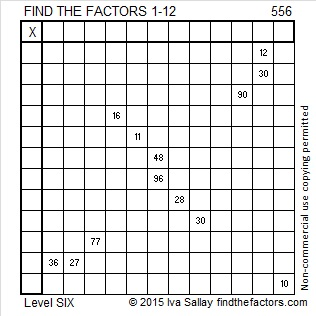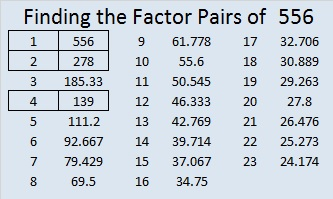# 556 and Level 6

556 is the sum of all the prime numbers from 131 to 149. One of those consecutive primes is also a prime factor of 556.Print the puzzles or type the solution on this excel file: 12 Factors 2015-07-13

—————————————————————————————————

• 556 is a composite number.
• Prime factorization: 556 = 2 x 2 x 139, which can be written 556 = (2^2) x 139
• The exponents in the prime factorization are 2 and 1. Adding one to each and multiplying we get (2 + 1)(1 + 1) = 3 x 2  = 6. Therefore 556 has exactly 6 factors.
• Factors of 556: 1, 2, 4, 139, 278, 556
• Factor pairs: 556 = 1 x 556, 2 x 278, or 4 x 139
• Taking the factor pair with the largest square number factor, we get √556 = (√4)(√139) = 2√139 ≈ 23.57965—————————————————————————————————This site uses Akismet to reduce spam. Learn how your comment data is processed.